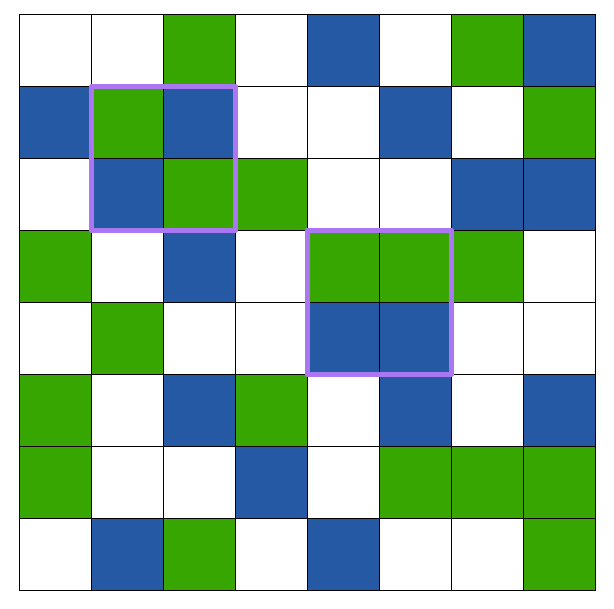# Can We Use Parity?

Probability Level 2Consider an $8 \times 8$ grid of squares. We color every square blue or green such that 3 of the 4 squares on the corners of the grid are blue and the fourth is green.

Will there always exist a $2 \times 2$ square inside this grid such that there are an odd number of squares of each color inside?

Bonus: Generalize this for the $n \times n$ grid.

×